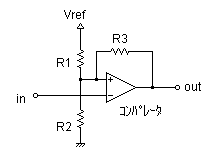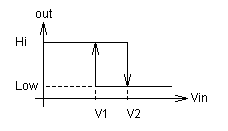# Comparator circuits with Hysteresis Design Tool

This page is a web application that design a comparator circuit with hysteresis. Use this utility to find the optimum resistors for hysteresis circuit from the resistor sequence.

## for a open collector(drain) output of comparatorSelect Resistor Sequence:
 Vref :V V1 :V V2 :V Vout(Low) :V (comparator output at saturation Low voltage)

## for a push-pull output of comparatorSelect Resistor Sequence:
 Vref :V V1 :V V2 :V Vout(Hi) :V (comparator output at saturation Hi voltage) Vout(Low) :V (comparator output at saturation Low voltage)

## Hysteresis voltage calculationR1 = [Ω] R2 = [Ω] R3 = [Ω]

 Vref = [V] Vout(Lo) = [V] Vout(Hi) = [V](for a push-pull output)

### Output type

open collector(drain)     push-pull

V1
V2